## 数字图像处理第1次实验实验报告

2806 15 2018年11月11日

0 0 投票数学校 华东师范大学 专业 计算机科学与技术 课程名称 数字图像处理 教师 童卫青 年份 2018年秋

## 一、实验目的

1. 了解 OpenCV 是如何使用的。
2. 了解在 C 语言程序中调用 OpenCV 进行图像的读、写、显示。
3. 了解图像的平移变换、放缩变换、旋转、剪切、转化为灰度等操作。
4. 掌握要完成图像的变化，应该用怎么样的矩阵对图像的像素进行操作。

## 二、实验内容

1. 配置运行环境、导入工程并运行程序，观察结果。
2. 理解代码，理解每一个函数的声明、作用、调用方法。
3. 了解图像的平移变换、放缩变换、旋转、剪切、转化为灰度等操作。

## 三、实验原理说明

### 3.1 工程的建立、OpenCV库的导入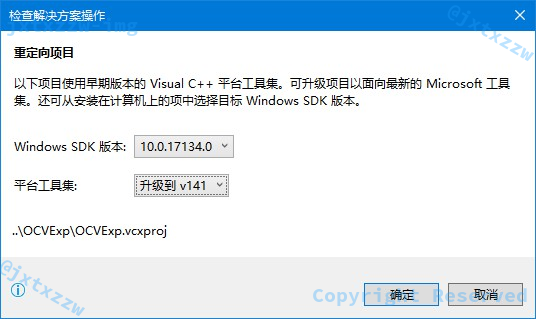OpenCV 库使用的是项目解决方案的动态链接库，而不是环境变量中的 OpenCV 库，所以理论上就算自己不安装 OpenCV 库也是完全可以使用的，配置信息都在项目文件里写好了。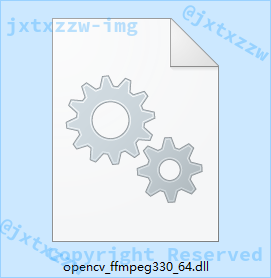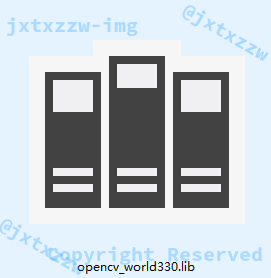### 3.2 一些基本信息的说明

#include <stdio.h>
#include "opencv.hpp"
using namespace std;
using namespace cv;

### 3.4 等待按键

cvWaitKey()是 OpenCV 的一个函数，他会阻塞自己，直到等待到一个按键。

### 3.6 图像的存储方式

Mat 类是一个 $n$ 维密集阵列。

Mat本质上是由两个数据部分组成的类：

• 包含信息有矩阵的大小，用于存储的方法，矩阵存储的地址等的矩阵头
• 包含了像素值的矩阵（可根据选择用于存储的方法采用任何维度存储数据）的一个指针

img1 = imread(fn);

// 拷贝图像
img2 = img1.clone();

img1 = img2;

## 四、函数使用说明

Mat imread(const String& filename, int flags=IMREAD_COLOR )

filename是要加载的文件的名称。

flag是指定加载图像的颜色类型的标志。如果不指定类型，那么默认是IMREAD_COLOR

• CV_LOAD_IMAGE_ANYDEPTH如果置位，当输入具有相应深度时返回 $16$ 位/ $32$ 位图像，否则将其转换为 $8$ 位。
• CV_LOAD_IMAGE_COLOR

如果设置，则始终将图像转换为彩色图像。

• CV_LOAD_IMAGE_GRAYSCALE

如果设置，则始终将图像转换为灰度图像。

• 如果值大于 $0$，返回三通道彩色图像。
• 需要特别说明的是，在当前实现中，会从输出图像中去除 Alpha 通道（如果有的话），如果需要 Alpha 通道，请使用负值。
• 如果值等于 $0$，返回灰度图像。
• 如果值小于 $0$，按原样返回加载的图像（使用Alpha通道）。

• Windows位图 – * .bmp， * .dib
• JPEG文件 – * .jpeg， * .jpg， *。jpe
• JPEG 2000文件 – * .jp2
• 便携式网络图形 – * .png
• WebP – * .webp
• 便携式图像格式 – * .pbm， * .pgm， * .ppm
• 太阳栅格 – * .sr， * .ras
• TIFF文件 – * .tiff， * .tif

// 读图像文件
cout << "读图像" << endl;

fn = "image\\lena.jpg";

// 拷贝图像
img2 = img1.clone();

### imwrite

bool imwrite(const String& filename, InputArray img, const vector<int>& params=vector<int>())

filename是文件的名称。

image是要保存的图像。

• 对于JPEG，它可以是从 $0$ 到 $100$ 的质量（CV_IMWRITE_JPEG_QUALITY）（越高越好）。
• 默认值为 $95$。
• 对于WEBP，它可以是从 $1$ 到 $100$ 的质量（CV_IMWRITE_WEBP_QUALITY）（越高越好）。
• 默认情况下（不带任何参数），如果质量高于 $100$，则使用无损压缩。
• 对于PNG，它可以是从 $0$ 到 $9$ 的压缩级别（CV_IMWRITE_PNG_COMPRESSION）。
• 值越大意味着更小的尺寸和更长的压缩时间。默认值为 $3$。
• 对于PPM，PGM或PBM，它可以是二进制格式标志（CV_IMWRITE_PXM_BINARY），$0$ 或 $1$。
• 默认值为 $1$。

//写图像文件
cout << "写图像" << endl;
fn = "img2.jpg";
imwrite(fn, img2);

### namedWindow与moveWindow

void namedWindow(const string＆winname，int flags = WINDOW_AUTOSIZE)

name是窗口标题中可用作窗口标识符的窗口的名称。

flags是窗口支持的标志。

• WINDOW_NORMAL如果设置了此项，则用户可以调整窗口大小（无约束）。
• WINDOW_AUTOSIZE

如果设置了此项，则会自动调整窗口大小以适合显示的图像，并且无法手动更改窗口大小。

• WINDOW_OPENGL

如果设置了此项，将使用 OpenGL 支持创建窗口。

void moveWindow(const string＆winname，int x，int y )

winname是窗口名称。

xy分别是窗口的新的x坐标和y坐标。

//创建图像显示窗口
namedWindow("image", CV_WINDOW_AUTOSIZE);
moveWindow("image", 0, 0);

### imshow

void imshow(const string＆winname，InputArray mat)

winname是窗口名称。

mat是要显示的图像。

• 如果图像是 $8$ 位无符号，则按原样显示。
• 如果图像是 $16$ 位无符号或 $32$ 位整数，则像素除以 $256$，即，值范围 $[0,255 \times 56]$ 被映射到 $[0,255]$。
• 如果图像是 $32$ 位浮点，则像素值乘以 $255$，即，值范围 $[0,1]$ 被映射到 $[0,255]$。

//显示图像
cout << "显示图像" << endl;
imshow("image",img2);
cvWaitKey(0);//等待按键

### waitKey

int waitKey(int delay = 0) //C++
int cvWaitKey(int delay = 0) //C

delay是以毫秒为单位的延迟。

$0$ 是特殊值，表示“永远”，此时直到按下鼠标按键或者按下键盘按键才会结束。

### cvtColor

void cvtColor(InputArray src, OutputArray dst, int code, int dstCn=0 )

src是输入图像即要进行颜色空间变换的原图像，可以是 Mat 类。

dst是输出图像即进行颜色空间变换后存储图像，也可以是 Mat 类 。

code是转换的代码或标识，即在此确定将什么制式的图片转换成什么制式的图片。

dstCn是目标图像通道数，如果取值为 $0$，则由srccode决定。默认值为 $0$。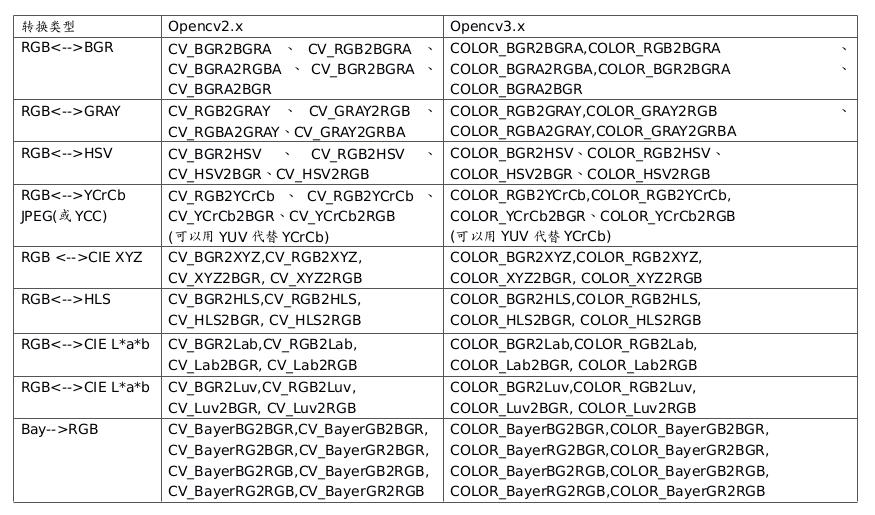CV_RGB2GRAY使用的是$\displaystyle I = \alpha \times R + \beta \times G + \gamma \times B$。

// 彩色图像转换为灰度图像
cout << "转换为灰度图像" << endl;
Mat grayImage;
cvtColor(img1, grayImage, CV_RGB2GRAY);
imshow("image", grayImage);
cvWaitKey(0);

### 变换矩阵

Mat 是 OpenCV 的一个类，表示一个矩阵。

• Mat (int rows, int cols, int type)
• Mat (Size size, int type)
• Mat (int rows, int cols, int type, const Scalar &s)
• Mat (Size size, int type, const Scalar &s)
• Mat (int ndims, const int *sizes, int type)
• Mat (int ndims, const int *sizes, int type, const Scalar &s)
• Mat (const Mat &m)
• Mat (int rows, int cols, int type, void *data, size_t step=AUTO_STEP)
• Mat (Size size, int type, void *data, size_t step=AUTO_STEP)
• Mat (int ndims, const int *sizes, int type, void *data, const size_t *steps=0)
• Mat (const Mat &m, const Range &rowRange, const Range &colRange=Range::all())
• Mat (const Mat &m, const Rect &roi)
• Mat (const Mat &m, const Range *ranges)

Mat (int rows, int cols, int type)

rowscols分别表示矩阵的行数和列数。

type表示矩阵的值的类型。

Mat am(2,3,CV_32FC1);// 2×3变换矩阵

am.at<float>(0, 0) = 1;

at方法接收一个范型的参数，表示数据的数据类型，在上面的代码中，这个数据是float类型的。

am.at<float>(0, 0) = 1;am.at<float>(0, 1) = 0;am.at<float>(0, 2) = 10;
am.at<float>(1, 0) = 0;am.at<float>(1, 1) = 1;am.at<float>(1, 2) = 10;

### warpAffine

void warpAffine(InputArray src, OutputArray dst, InputArray M, Size dsize, int flags=INTER_LINEAR, int borderMode=BORDER_CONSTANT, const Scalar& borderValue=Scalar())

src是输入图像。

dst是输出具有dsize大小和与src类型相同的图像。

M是一个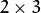的变换矩阵。

dsize是输出图像的大小。

flags是插值方法的组合和可选的标记WARP_INVERSE_MAP，表示这M是逆变换（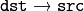）。

borderMode表示像素外推法，当 borderMode=BORDER_TRANSPARENT，这意味着该功能不会修改与源图像中的异常值对应的目标图像中的像素。

borderValue表示在边界不变的情况下使用的值。默认情况下，它为0。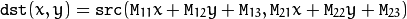warpAffine(img1, img2, am, img2.size());

### cvDestroyWindow

void destroyWindow(const string& winname)

winname是需要销毁的窗口的名称。

cvDestroyWindow("image");

## 五、实验结果及分析

### 彩色图像转换为灰度图像

// 彩色图像转换为灰度图像
cout << "转换为灰度图像" << endl;
Mat grayImage;
cvtColor(img1, grayImage, CV_RGB2GRAY);
imshow("image", grayImage);
cvWaitKey(0);

cvtColor会对img1的每一个像素的RGB三个通道进行 $\displaystyle I = 0.299 \times R + 0.587 \times G + 0.114 \times B$ 运算，并将计算后的值作为新的像素点的灰度值。### 图像平移变换

am.at<float>(0, 0) = 1;am.at<float>(0, 1) = 0;am.at<float>(0, 2) = 10;
am.at<float>(1, 0) = 0;am.at<float>(1, 1) = 1;am.at<float>(1, 2) = 10;

warpAffine(img1, img2, am,img2.size());
imshow("image", img2);
cvWaitKey(0);### 图像缩小变换

am.at<float>(0, 0) = 0.5F; am.at<float>(0, 1) = 0.0F; am.at<float>(0, 2) = 0;
am.at<float>(1, 0) = 0.0F; am.at<float>(1, 1) = 0.5F; am.at<float>(1, 2) = 0;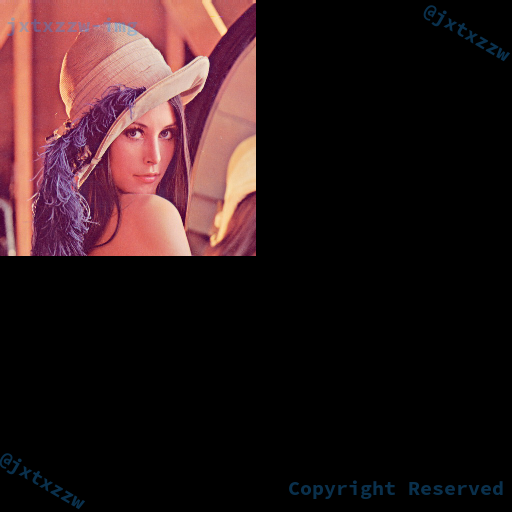### 图像放大变换

am.at<float>(0, 0) = 1.5F; am.at<float>(0, 1) = 0.0F; am.at<float>(0, 2) = 0;
am.at<float>(1, 0) = 0.0F; am.at<float>(1, 1) = 1.5F; am.at<float>(1, 2) = 0;### 图像旋转变换

float angle = 30;

float phi = (float)(angle*CV_PI / 180.0F);

float angle = 30;
int cenx = 256;
int ceny = 256;
float phi = (float)(angle*CV_PI / 180.0F);
am.at<float>(0, 0) = (float)cos(phi); am.at<float>(0, 1) = -(float)sin(phi); am.at<float>(0, 2) = (float)(cenx - cenx * cos(phi) + ceny * sin(phi));
am.at<float>(1, 0) = (float)sin(phi); am.at<float>(1, 1) = (float)cos(phi); am.at<float>(1, 2) = (float)(ceny - cenx * sin(phi) - ceny * cos(phi));float angle = 45;
int cenx = 100;
int ceny = 200;
float phi = (float)(angle*CV_PI / 180.0F);
am.at<float>(0, 0) = (float)cos(phi); am.at<float>(0, 1) = -(float)sin(phi); am.at<float>(0, 2) = (float)(cenx - cenx * cos(phi) + ceny * sin(phi));
am.at<float>(1, 0) = (float)sin(phi); am.at<float>(1, 1) = (float)cos(phi); am.at<float>(1, 2) = (float)(ceny - cenx * sin(phi) - ceny * cos(phi));### 图像剪切变换

am.at<float>(0, 0) = 1; am.at<float>(0, 1) = 0.3F; am.at<float>(0, 2) = 0;
am.at<float>(1, 0) = 0; am.at<float>(1, 1) = 1.0F; am.at<float>(1, 2) = 0;am.at<float>(0, 0) = 0.5F; am.at<float>(0, 1) = 0.3F; am.at<float>(0, 2) = 0;
am.at<float>(1, 0) = 0; am.at<float>(1, 1) = 0.8F; am.at<float>(1, 2) = 0;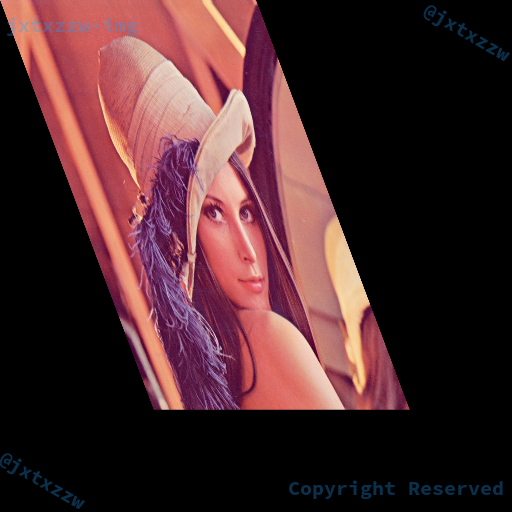## 六、更多尝试与思考

### 灰度变换

int gray = (int) (0.299 * c.getRed() + 0.587 * c.getGreen() + 0.114 * c.getBlue());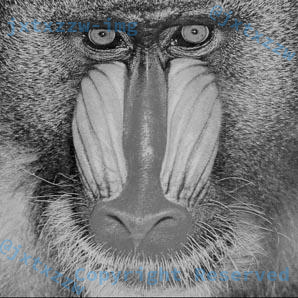### 剪切变换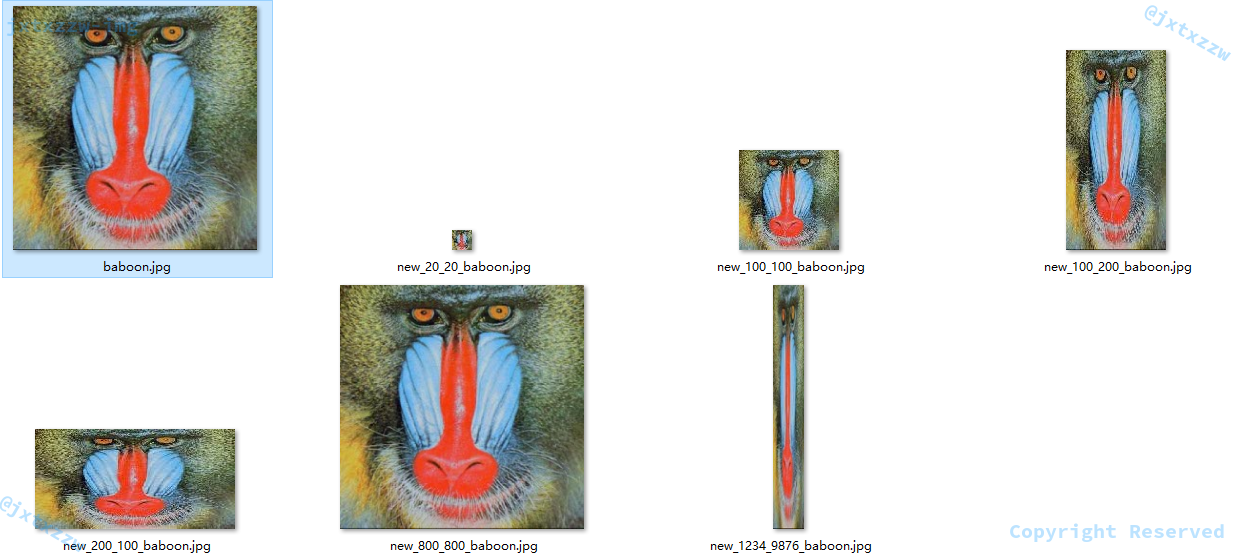### Blur 滤镜（模糊）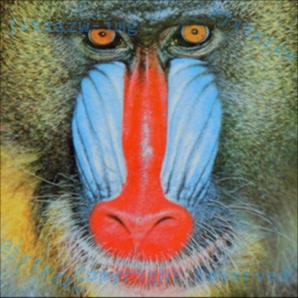### Sharpen 滤镜（锐化）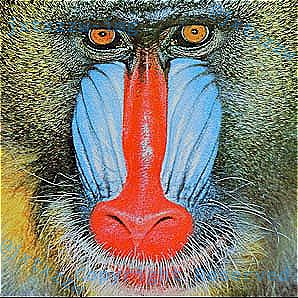### Linear 滤镜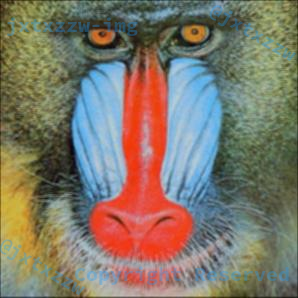### Edge 滤镜（取边缘）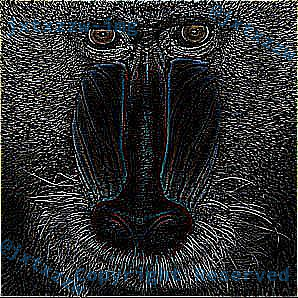### Emboss 滤镜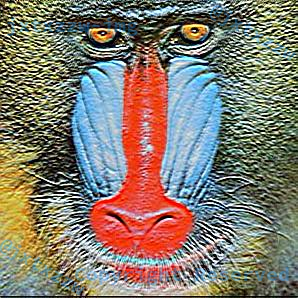### Oil 滤镜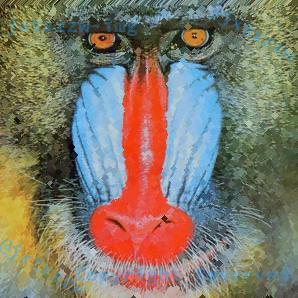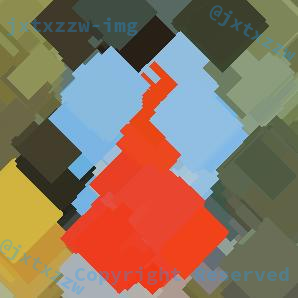for (int col = 0; col < pin.width(); col++) {
for (int row = 0; row < pin.height(); row++) {
HashMap<Color, Integer> freq = new HashMap<Color, Integer>();
Color t = null;
int max = 0;
for (int i = row - w; i < row + w; i++) {
for (int j = col - w; j < col + w; j++) {
if (i >= 0 && i < pin.height() && j >= 0 && j < pin.width()
&& Math.abs(row - i) + Math.abs(col - j) < w) {
Color c = pin.get(j, i);
if (freq.containsKey(c)) {
freq.put(c, freq.get(c) + 1);
if (freq.get(c) > max) {
max = freq.get(c);
t = new Color(c.getRed(), c.getGreen(), c.getBlue());
}
} else {
freq.put(c, 1);
if (freq.get(c) > max) {
max = freq.get(c);
t = new Color(c.getRed(), c.getGreen(), c.getBlue());
}
}
}
}
}
pout.set(col, row, t);
}
}
0 0 投票数

15条留言（可选）如果您也有个人网站，不妨分享一下

15 评论kk23d (@guest_2445)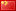Chrome 84.0.4147.125 Mac OS X 10_14_5

2020年8月21日 03:14

tqlQQ浏览器 10.5.3866.400 Windows 10

2020年5月4日 11:14Chrome 80.0.3987.163 Windows 10

2020年4月6日 17:31Firefox 71.0 Windows 10

2019年12月9日 09:40_(@_)Chrome 77.0.3865.120 Windows 10
2019年10月21日 01:04

tqlkoko (@guest_810)Chrome 77.0.3865.120 Windows 10

2019年10月20日 21:32

QAQlcatastrophe (@guest_808)Edge 18.17763 Windows 10

2019年10月20日 16:22Firefox 69.0 Windows 10
2019年10月19日 20:02

QWQFirefox 66.0 Windows 10

2019年5月2日 09:04Emily (@guest_291)Safari 605.1.15 Mac OS X 10_14

2019年3月3日 23:06

QAQ